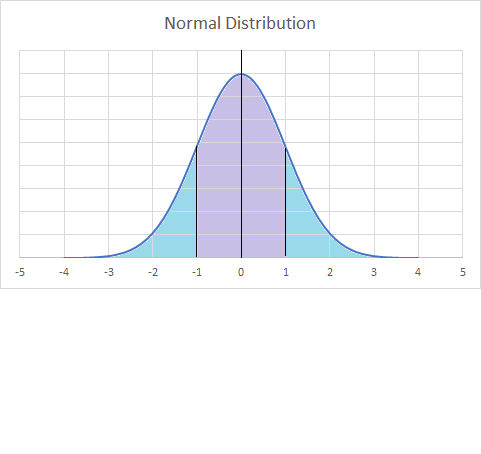# Definition of Normal DistributionA normal distribution is a common way for continuous data to be distributed.

The normal distribution is a "bell curve" like the one in the picture.

Even if data doesn't exactly follow the normal distribution, the normal distribution can often be used to approximate the data.

A number of different types of data follow a normal distribution:

• The heights and weights of people.
• Measurement errors.
• The lengths of lizards.
• The widths of planks produced by a machine.

You will often hear teachers talking about "curving" the results of a test. By this, they mean that they will change the test marks so that they follow a normal distribution.

### Description

The aim of this dictionary is to provide definitions to common mathematical terms. Students learn a new math skill every week at school, sometimes just before they start a new skill, if they want to look at what a specific term means, this is where this dictionary will become handy and a go-to guide for a student.

### Audience

Year 1 to Year 12 students

### Learning Objectives

Learn common math terms starting with letter N

Author: Subject Coach
You must be logged in as Student to ask a Question.